## Recent Posts

1. If 520 mangoes can be bought for Rs. 600, how many can be bought for Rs. 1500?
[a] 1040
[b] 1200
[c] 1300
[d] 15602. In an examination 86% of the candidates passed and 224 failed. How many candidates appeared for the exam?
[a] 800
[b] 1540
[c] 1600
[d] 31363. Raju and Tom together can do a work in 10 days. Tom & Appu together can do it in 12 days. Appu and Raju together can do it in 15 days. In how many days will they finish it if all the tree work together?
[a] 8 days
[b] 4 days
[c] 4.5 days
[d] 9.5 days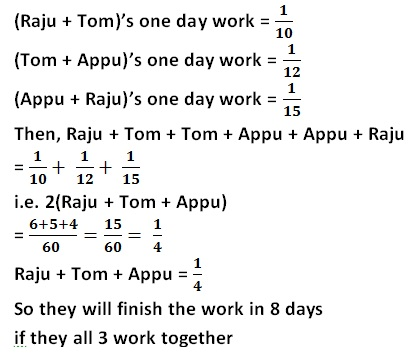4. If Maya goes to office at a speed of 40 km/hr. She reaches 5 minutes late. If she travels at the speed of 60 km/hr. She is 10 minutes early. What is the distance to office from her home?
[a] 30 km
[b] 40 km
[c] 50 km
[d] 35 km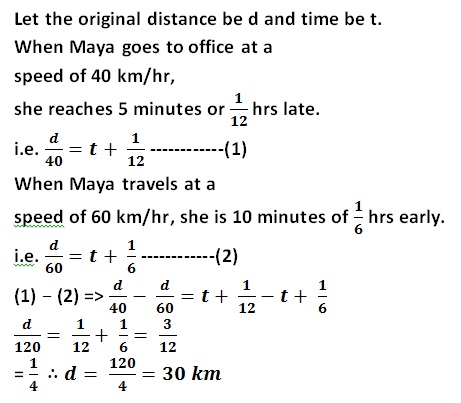5. How much time will a train of length 171 meters take to cross a tunnel of length 229 meters, if it is running at a speed of 30 km/hr?
[a] 30 seconds
[b] 36 seconds
[c] 48 seconds
[d] 60 seconds6. If Today 10th May 2018 is Thursday. What day of the week will it be on 25th December 2018?
[a] Wednesday
[b] Sunday
[c] Monday
[d] Tuesday7. The simple interest on a sum of money is equal to the principal and number of years is equal to the rate percent per annum. Find the rate percent.
[a] 15%
[b] 20%
[c] 12%
[d] 10%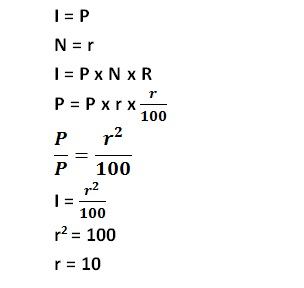8. How long will a 150m long train running at a speed of 60 kmph takes to cross the bridge of 300m long?
[a] 25 seconds
[b] 27 seconds
[c] 35 seconds
[d] 37 seconds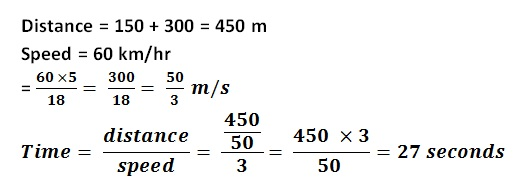9. The ratio of ages of Monu and Sonu at present is 4:1. Eight years ago Manu’s age was 10 times the age of Sonu. What is the present age of Monu?
[a] 48
[b] 44
[c] 46
[d] 42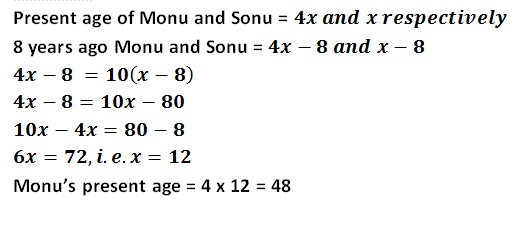10. The ratio between the ages of Appu and Ryan at present is 3:4. Five years ago the ratio of their ages was 2:3. What is the present age of Appu?
[a] 15 years
[b] 20 years
[c] 12 years
[d] 30 yearsKerala PSC GK | Practice/Model Maths Questions - 1Reviewed by Santhosh Nair on 2:38 AM Rating: 5